• 本书以严谨的思路、灵活的方式讲述了高等院校线性代数与解析几何课程的内容，既突出了线性代数作为各专业公共课程的工具性操作性，也反映了线性代数与解析几何、多项式知识的思想性以及它们之间的联系。...
•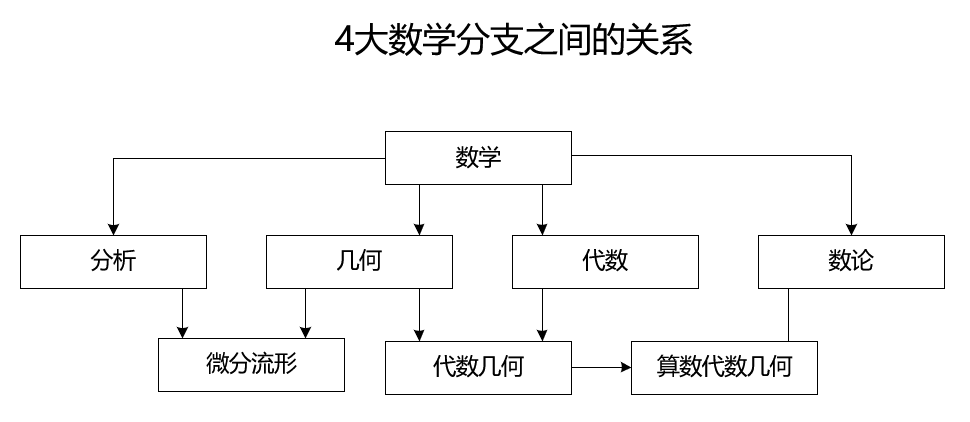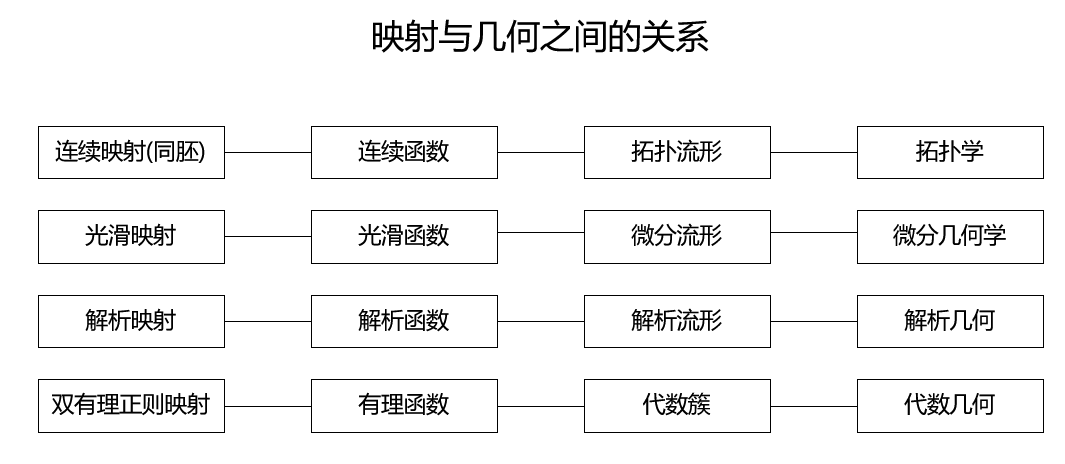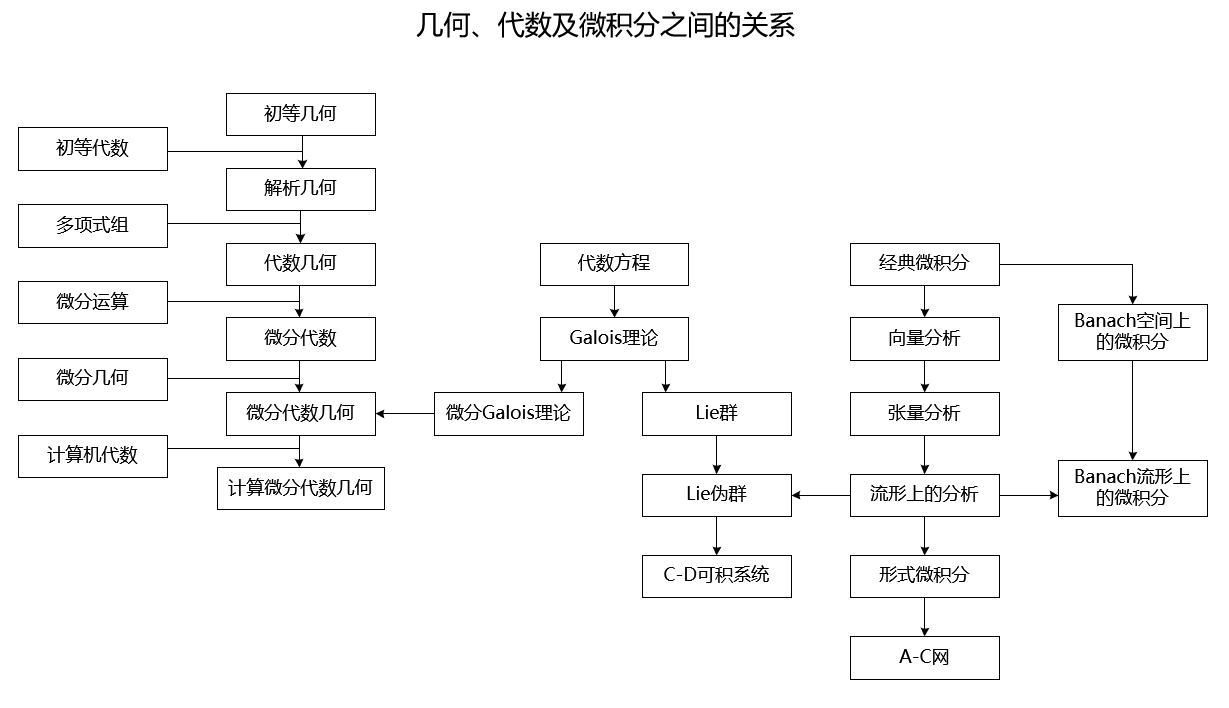展开全文微分流形
• 线性代数几何意义矩阵的几何意义矩阵由若干向量组成（可以是有限个，也可以是无限可数个），其形式数学史赋予它的最自然的几何含义线性空间有关（向量间的加法以及另一个数集带来的乘法为这个空间赋予了基本...
直到今天看到这个网页，才看见有人一语道破线性代数的真谛（这也是我终于决定写成此文的直接原因）。我终于找到了我那一个学期企图寻找的东西。就好像把 x 变成 2 x 一样，我们经常需要把 (x, y) 变成 (2 x + y, x - 3 y) 之类的东西，这就叫做线性变换。于是才想到定义矩阵乘法，用于表示一切线性变换。几何上看，把平面上的每个点 (x, y) 都变到 (2 x + y, x - 3 y) 的位置上去，效果就相当于对这个平面进行了一个“线性的拉扯”。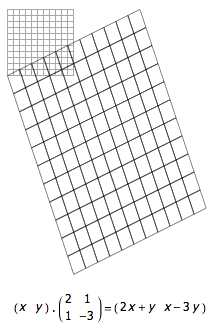矩阵的乘法，其实就是多个线性变换叠加的效果，它显然满足结合律，但不满足交换律。主对角线全是 1 的矩阵所对应的线性变换其实就是不变的意思，因此它叫做单位矩阵。矩阵 A 乘以矩阵 B 得单位矩阵，就是做完线性变换 A 后再做一次线性变换 B 就又变回去了的意思，难怪我们说矩阵 B 是矩阵 A 的逆矩阵。课本上对行列式的定义千奇百怪，又是什么递归，又是什么逆序对，还编写口诀帮助大家记忆。其实，行列式的真正定义就一句话：每个单位正方形在线性变换之后的面积。因此，单位矩阵的行列式当然就为 1，某行全为 0 的行列式显然为 0 （因为某一维度会被无视掉，线性变换会把整个平面压扁）， |A·B| 显然等于 |A|·|B| 。行列式为 0 ，对应的矩阵当然不可逆，因为这样的线性变换已经把平面压成一条线了，什么都不能把它变回去了。当然，更高阶的矩阵就对应了更高维的空间。一瞬间，所有东西都解释清楚了。
几何意义
线性代数的几何意义矩阵的几何意义矩阵由若干向量组成（可以是有限个，也可以是无限可数个），其形式和数学史赋予它的最自然的几何含义和线性空间有关（向量间的加法以及另一个数集带来的乘法为这个空间赋予了基本结构），这部分内容将在后续更新里单独列出来讲。这里不妨先简单直观一些，要么把矩阵画成几个行向量或列向量，要么画成由向量终点组成的图形，这刚好和当代计算机图形学有联系，例如大家常玩的3D游戏或某些基于矢量绘图引擎的2D游戏，就都是矩阵可视化以及矩阵变换的生动实例。如矩阵 按照列向量可表示为如下图形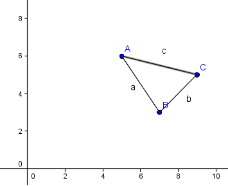如下图是在matlab中将z=sin(x)*cos(y)算得的离散点组成的矩阵表示成几何图形，当你旋转这个图形观察时，每个画面都是计算机用相应的旋转和投影变换矩阵，对原始的图形数据矩阵相乘变换后得到的，在3D游戏中移动、转动、缩放和光照等也都是靠矩阵运算完成的，当然这里让我们初步感受到一种魔力——矩阵既可以用来表示纯数据（如复杂图形的顶点），也可以用来对数据做变换，在以后的学习中我们会看到，这其实是在说，不仅某阶向量和矩阵全体可以构成一个线性空间，它上面的全体线性变换也构成一个线性空间，即任何线性变换都可以在选择确定的基后，用矩阵来表示。但神奇的东西何止于此，背后还隐藏着更深刻的内容——借用语言哲学的思想，我们对数学语言本身进行反思，会有诸多更本质的东西显现，例如你开始可能会以为离散的加减乘除运算包括矩阵运算等，在工程应用时只能近似和将就，以为偏微分方程等基于连续性的微积分工具才是宝典，但最终会发现，我们所拥有的原子运算只有基础代数运算，而真正"存在"的数学对象都是离散的（连续是一种幻象，或者说只是一个语言概念，而且充当这个语言里的相对本体，可参见奎因哲学），数学世界乃至物理世界都是由离散的对象和它们之间的关系所定义的，这方面有兴趣可以去看看代数几何与前沿物理的思想。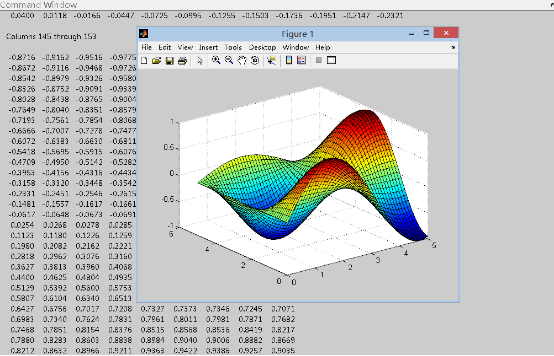注1：如果单独查看一个矩阵 可以有两种解读：矩阵A由m个n维向量组成，或者由n个m维向量组成；在使用时会根据实际情或约定选择其中一种，而在参与变换或其他运算时，这两种解读一般不能混淆，一定要确定注2：当我们把矩阵表示成图形时，其作图没有固定标准，并不一定是把所有向量终点连接起来构成一个多边形，规则是使用者制定的，可以是网格，可以是离散面片等行列式的几何意义方阵 的行列式的绝对值是其行向量或列向量所张成的平行几何体的空间积，对于二阶行列式，就是向量张成的平行四边形的面积，对于三阶行列式，就是对应平行六面体的体积；如方阵 的行列式绝对值为27，它就是下图平行四边形的面积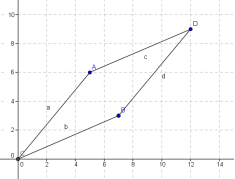注：行列式其实是带有符号的，实际上，正负号表征了这些向量作为线性空间基的手性，正号表示右手系，负号表示左手系，在二阶矩阵的向量空间里，其判别方法是，伸出右手和矩阵的第一个列向量或行向量平行，然后调整手的正反使得能从此向量转过小于180度的角到达第二个向量，这时大拇指如果朝上（从纸面指向自己）则为右手系，矩阵的行列式为正，反之则为左手系，对应行列式为负；如果是三阶矩阵，则从第一个向量转向第二个向量时，如果大拇指指向第三个向量方向（不必重合），则为右手系，其行列式为正，反之为左手系，行列式为负；其实这一点上更广义的表述应是向量空间的基相对自然坐标系的顺序性（代数上可用逆序数表达）克拉默法则的几何意义以二维形式为例来说明其几何意义:现有方程： 其中 ，记 ， 原方程也可表示为 这样可以把 与 看作是列向量 和 的缩放因子，经过伸缩后再叠加即得到和向量 ，故原方程可以解读为把A的列向量缩放并叠加后得到向量 ，求伸缩因子 我们已经知道行列式的几何意义，显然矩阵A对应的平行四边形的面积就是|A|（这里以带符号的有方向面积表示，因为伸缩因子也是有符号的），当某一个向量被伸缩后，如图将OB边伸长至OE，形成新的平行四边形OAFE，记其面积为 这样 的伸缩因子 可表示为 所以只要求出OAFE的面积即可解出未知量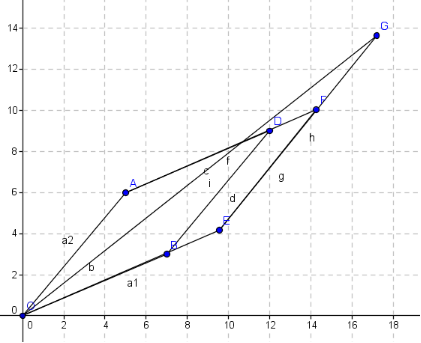图中OG即向量b，因为它是 的线性叠加，所以G点必在EF的延长线上，这样OG和OE相对OA边的高就是相同的，故OA与OG组成的平行四边形面积和OAFE相同，即所求面积为 ，所以 ，同理可得 矩阵乘法的几何意义我们知道矩阵是由若干向量组成的，因此可自然地把矩阵乘法看作是两个矩阵的同维向量之间做内积（或点乘），而内积的意义是两向量同向投影的乘积，但这只是一个表面的几何含义，比较抽象（也有应用之处，后面会提到）；实际上，对于矩阵乘法C=AB，作用后得到的新矩阵C可以看作是矩阵A经过某种变换得到的，也可以看作是矩阵B经过某种变换后得到的，而这种变换显然就是乘以另一个矩阵的过程，结合前面提到的矩阵的几何意义，故可以把矩阵乘法C=AB看作是图形A（或B）经过变换B（或A）后得到新图形C，或者是向量空间A（或B）经过变换B（或A）后得到新的向量空间C，对于简单的变换矩阵这一点最容易感性体会到；例如变换矩阵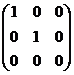会把原3D图形向x-y面投影，变换矩阵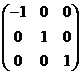会把原图形对x轴镜像，变换矩阵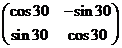会把原2D图形相对原点逆时针旋转30度。初等变换的几何意义由前面叙述的部分几何意义，我们很快就能看出初等变换的几何含义了交换矩阵的两行（列）：改变向量在矩阵中的排列顺序，当矩阵表示图形时，此操作对图形没有影响，因而矩阵张成的空间维数（秩）不变，但是当矩阵代表向量空间时，会改变此坐标系的手性，当计算方阵的行列式时，会改变其符号；以一个非零数k乘矩阵的某一行（列）：即对矩阵中某一向量进行伸缩变换，整个矩阵代表的图形对应发生变化，由于k不能为0，所以矩阵张成空间的维数（秩）不变，方阵张成的平行几何体的空间积（行列式）变成原来的k倍把矩阵的某一行（列）的k倍加于另一行（列）上：对矩阵中某一向量做线性叠加，且新向量终点总是在另一向量的平行线上，所以对任意矩阵，图形产生了剪切变形，由于剪切变形不会使向量重叠或缩为0，所以张成空间的维数也不变；对于方阵，由前面几何推导克拉默法则的过程知道，如果把某一向量加上矩阵内另一向量的k倍，由于新向量和原向量相对其余向量组成的平行体的高不变，所以方阵对应的平行几何体的空间积不变（行列式不变），例如在matlab中用矩阵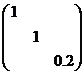作用下面左图对应的矩阵（第三行乘以0.2，即缩短z方向坐标5倍），得到的新图形如下右图所示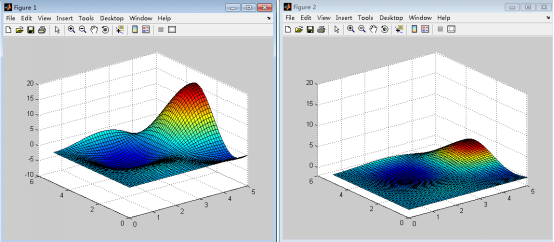Matlab程序如下，可以动手试一试，还可修改其中的变换矩阵以得到不同效果x=0:0.1:5;y=x;[x y]=meshgrid(x,y); %构造网格z=sin(x).cos(y).x.y; surf(x,y,z); %绘制原图形x=reshape(x,2601,1);y=reshape(y,2601,1);z=reshape(z,2601,1);m=[x y z]; %几何图形对应的n3矩阵t=[1 0 0;0 1 0;0 0 0.2]; %变换矩阵m=mt; %进行变换x=m(:,1);y=m(:,2);z=m(:,3);x=reshape(x,51,51);y=reshape(y,51,51);z=reshape(z,51,51);figure;surf(x,y,z) %绘制变换后的图形然后我们把变换矩阵修改为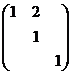即把第二行乘以2加到第一行，由上述分析知道这样会把原图形沿y方向剪切变形，剪切量为对应x坐标的二倍，实际效果如下图所示，这里我们取俯视角以观察x-y面的情形，从右图可以看出理论分析是正确的（注意观察变换前后的y向坐标值）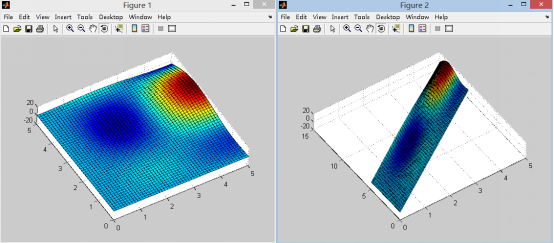矩阵秩的几何意义矩阵的秩即矩阵的各向量所张成空间的维数不能说秩是矩阵对应图形的维数，因为矩阵的图形只取了各向量的终点，而不含有这些向量的之间的几何关系，故二者的维数不一定相等，而矩阵的秩按定义应取其向量空间维数。如下图中的空间向量a,b,c可以张成一个三维空间，故矩阵(a b c)的秩为3，但是其终点组成的图形是一平面，维数为2，显然和秩是不一样的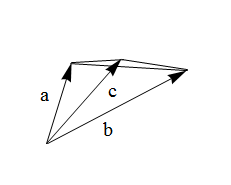结合上面对初等变换的几何解释，正是因为三种初等变换都不改变矩阵向量空间的维数，所以对于复杂的难以观察维数的矩阵，我们可以先用初等变换作用于矩阵进行简化，然后到容易观察的形式时求出它的秩；向量组线性相关/无关的几何意义注：在讨论向量张成的空间相关问题时，某种程度上我们可以把向量组和矩阵等价对待，二者都是一组向量的集合，只是向量组相对矩阵明确了向量的维数与向量个数，而矩阵有行与列两种选择，所以只要确定矩阵的向量取行还是列，就可以把矩阵当作向量组讨论；线性相关在代数上就是一组向量中至少有一个向量能用其余向量线性表示，而几何意义是它们所张成的向量空间维数少于这些向量的个数，这样就至少存在一个向量落在其余向量形成的向量空间中，而向量空间实际上是一个坐标系统，所以处于其中的点（向量）都可以由这些向量定位出来（线性表示），在向量之间表现出一种相关性；而线性无关的几何意义就是一组向量张成空间的维数等于这些向量的个数，这样没有任何一个向量落在其余向量形成的空间里，每一个向量对其余向量来说都是超越自身空间维度的（独立的），因而无法被定位（线性表示），表现成一种相互无关性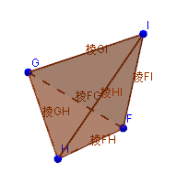以上图棱锥为例，因为HI处于GH和GI所形成的面里，所以HI必然可以由这两个向量表示，所以三者线性相关（三者形成的空间维数为2<3）；而HI在IG和IF形成的平面之外，所以H点无论如何都不能被GI和IF定位到，同时IF也不在IG和HI形成的平面里，IG不在IH和IF形成的平面里，同理可知它们之间不能线性表示，所以三者线性无关（三者形成的空间维数为3=向量个数）方程Ax=0的几何意义由前面叙述容易看出此方程表示向量x与A的每一个行向量都垂直，或者说向量x垂直于矩阵A的行向量空间。这样我们可以直接根据几何意义得到结论：Ax=0有非零解的充要条件是矩阵A的秩要小于x的维数n；这是因为对于确定维度的向量空间M，如果我们可以找出独立于它的一维或多维空间N，则在空间N里的向量总是垂直于空间M；例如在直角坐标系O-xyz中，设A是x-y平面上的向量空间，x是空间向量，因为z维上的向量总是垂直于A，所以x在这一方向上存在无数非零解。反之若矩阵A的秩等于n，且x非零，则由于x也在n维空间内，所以它和A中的行向量必然线性相关，无法独立于A的行向量空间，所以这时仅有零解。当方程有非零解时，设A的向量空间维数为R（秩），由上叙述可知解向量x中存在n-R个分量取值自由，如果我们把这n-R个自由变量看作是一个n-R维空间中的向量坐标时，显然此空间中每一个向量都能确定原方程组的一个解，又因为每一个向量都可以用这个n-R维空间的一组单位正交基线性表示，所以这组单位正交向量所确定的一组解通过线性组合就可以表示出原方程的任意解，故这组解就是原方程的一个基础解系，上述叙述也正是基础解系的几何意义方程Ax=b的几何意义设A是mn矩阵，x是n维向量，由前述几何意义知道，如果b处于A的向量空间中（b和A的向量线性相关），则一定可以由A的向量线性表示，也即解存在，而b落在A的向量空间等价于b的维数小于等于向量空间A的维数，也可表述为R(A)=R(A b)=R，即A的秩等于增广矩阵的秩，这种表达也是许多教科书中常用的。当R=n时，n维向量x的每个分量都是线性表示的确定系数，故只有唯一解，而R<n时，向量空间有n-R个维度不存在，故这些维度上对应的系数可任意（自由变量），这时存在无穷多解
展开全文• 数学发展到现在，已经成为科学世界中拥有100多个主要分支学科的庞大的“共和国”。大体说来，数学中研究数的部分属于代数学的范畴；研究形的部分，属于几何学的范筹；沟通形与数且...

数学发展到现在，已经成为科学世界中拥有100多个主要分支学科的庞大的“共和国”。大体说来，数学中研究数的部分属于代数学的范畴；研究形的部分，属于几何学的范筹；沟通形与数且涉及极限运算的部分，属于分析学的范围。这三大类数学构成了整个数学的本体与核心。在这一核心的周围，由于数学通过数与形这两个概念，与其它科学互相渗透，而出现了许多边缘学科和交叉学科。本章简要介绍数学三大核心领域中十几门主要分支学科的有关历史发展情况。

代数学范畴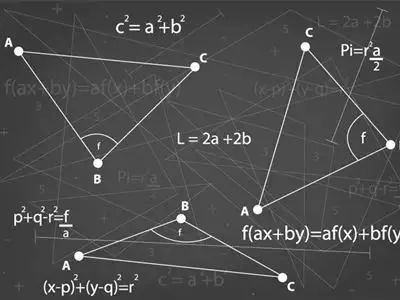1
算数

算术有两种含义，一种是从中国传下来的，相当于一般所说的“数学”，如《九章算术》等。另一种是从欧洲数学翻译过来的，源自希腊语，有“计算技术”之意。现在一般所说的“算术”，往往指自然数的四则运算；如果是在高等数学中，则有“数论”的含义。作为现代小学课程内容的算术，主要讲的是自然数、正分数以及它们的四则运算，并通过由计数和度量而引起的一些最简单的应用题加以巩固。
算术是数学中最古老的一个分支，它的一些结论是在长达数千年的时间里，缓慢而逐渐地建立起来的。它们反映了在许多世纪中积累起来，并不断凝固在人们意识中的经验。
自然数是在对于对象的有限集合进行计算的过程中，产生的抽象概念。日常生活中要求人们不仅要计算单个的对象，还要计算各种量，例如长度、重量和时间。为了满足这些简单的量度需要，就要用到分数。
现代初等算术运算方法的发展，起源于印度，时间可能在10世纪或11世纪。它后来被阿拉伯人采用，之后传到西欧。15世纪，它被改造成现在的形式。在印度算术的后面，明显地存在着我国古代的影响。
19世纪中叶，格拉斯曼第一次成功地挑选出一个基本公理体系，来定义加法与乘法运算；而算术的其它命题，可以作为逻辑的结果，从这一体系中被推导出来。后来，皮亚诺进一步完善了格拉斯曼的体系。
算术的基本概念和逻辑推论法则，以人类的实践活动为基础，深刻地反映了世界的客观规律性。尽管它是高度抽象的，但由于它概括的原始材料是如此广泛，因此我们几乎离不开它。同时，它又构成了数学其它分支的最坚实的基础。

2
初等代数

作为中学数学课程主要内容的初等代数，其中心内容是方程理论。代数一词的拉丁文原意是“归位”。代数方程理论在初等代数中是由一元一次方程向两个方面扩展的：其一是增加未知数的个数，考察由有几个未知数的若干个方程所构成的二元或三元方程组(主要是一次方程组)；其二是增高未知量的次数，考察一元二次方程或准二次方程。初等代数的主要内容在16世纪便已基本上发展完备了。
古巴比伦(公元前19世纪～前17世纪)解决了一次和二次方程问题，欧几里得的《原本》(公元前4世纪)中就有用几何形式解二次方程的方法。我国的《九章算术》(公元1世纪)中有三次方程和一次联立方程组的解法，并运用了负数。3世纪的丢番图用有理数求一次、二次不定方程的解。13世纪我国出现的天元术(李冶《测圆海镜》)是有关一元高次方程的数值解法。16世纪意大利数学家发现了三次和四次方程的解法。
代数学符号发展的历史，可分为三个阶段。第一个阶段为三世纪之前，对问题的解不用缩写和符号，而是写成一篇论文，称为文字叙述代数。第二个阶段为三世纪至16世纪，对某些较常出现的量和运算采用了缩写的方法，称为简化代数。三世纪的丢番图的杰出贡献之一，就是把希腊代数学简化，开创了简化代数。然而此后文字叙述代数，在除了印度以外的世界其它地方，还十分普通地存在了好几百年，尤其在西欧一直到15世纪。第三个阶段为16世纪以后，对问题的解多半表现为由符号组成的数学速记，这些符号与所表现的内容没有什么明显的联系，称为符号代数。16世纪韦达的名著《分析方法入门》，对符号代数的发展有不少贡献。16世纪末，维叶特开创符号代数，经笛卡尔改进后成为现代的形式。
“＋”、“－”号第一次在数学书中出现，是1489年魏德曼的著作。不过正式为大家所公认，作为加、减法运算的符号，那是从1514年由荷伊克开始的。1540年，雷科德开始使用现在使用“＝”。到1591年，韦达在著作中大量使用后，才逐渐为人们所接受。1600年哈里奥特创用大于号“＞”和小于号“＜”。1631年，奥屈特给出“×”、“÷”作为乘除运算符。1637年，笛卡尔第一次使用了根号，并引进用字母表中头前的字母表示已知数、后面的字母表示未知数的习惯做法。至于“≮”、“≯”、“≠”这三个符号的出现，那是近代的事了。
数的概念的拓广，在历史上并不全是由解代数方程所引起的，但习惯上仍把它放在初等代数里，以求与这门课程的安排相一致。公元前4世纪，古希腊人发现无理数。公元前2世纪(西汉时期)，我国开始应用负数。1545年，意大利的卡尔达诺开始使用虚数。1614年，英国的耐普尔发明对数。17世纪末，一般的实数指数概念才逐步形成。

3
高等代数

在高等代数中，一次方程组（即线性方程组）发展成为线性代数理论；而—、二次方程发展成为多项式理论。前者是向量空间、线性变换、型论、不变量论和张量代数等内容的一门近世代数分支学科，而后者是研究只含有一个未知量的任意次方程的一门近世代数分支学科。作为大学课程的高等代数，只研究它们的基础。
1683年关孝和(日本人)最早引入行列式概念。关于行列式理论最系统的论述，则是雅可比1841年的《论行列式的形成与性质》一书。在逻辑上，矩阵的概念先于行列式的概念；而在历史上，次序正相反。凯雷在1855年引入了矩阵的概念，在1858年发表了关于这个课题的第一篇重要文章《矩阵论的研究报告》。
19世纪，行列式和矩阵受到人们极大的关注，出现了千余篇关于这两个课题的文章。但是，它们在数学上并不是大的改革，而是速记的一种表达式。不过已经证明它们是高度有用的工具。
多项式代数的研究始于对3、4次方程求根公式的探索。1515年，菲洛解决了被简化为缺2次项的3次方程的求解问题。1540年，费尔拉里成功地发现了一般4次方程的代数解法。人们继续寻求5次、6次或更高次方程的求根公式，但这些努力在200多年中付诸东流。
1746年，达朗贝尔首先给出了“代数学基本定理”的证明(有不完善之处)。这个定理断言：每一个实系数或复系数的n次代数方程，至少有一个实根或复根。因此，一般地说，n次代数方程应当有n个根。1799年，22岁的高斯在写博士论文中，给出了这个定理的第一个严格的证明。1824年，22岁的阿贝尔证明了：高于4次的一般方程的全部系数组成的根式，不可能是它的根。1828年，年仅17岁的伽罗华创立了“伽罗华理论”，包含了方程能用根号解出的充分必要条件。

4
数论

以正整数作为研究对象的数论，可以看作是算术的一部分，但它不是以运算的观点，而是以数的结构的观点，即一个数可用性质较简单的其它数来表达的观点来研究数的。因此可以说，数论是研究由整数按一定形式构成的数系的科学。
早在公元前3世纪，欧几里得的《原本》讨论了整数的一些性质。他证明素数的个数是无穷的，他还给出了求两个数的公约数的辗转相除法。这与我国《九章算术》中的“更相减损法”是相同的。埃拉托色尼则给出了寻找不大于给定的自然数N的全部素数的“筛法”：在写出从1到N的全部整数的纸草上，依次挖去2、3、5、7……的倍数(各自的2倍，3倍，……)以及1，在这筛子般的纸草上留下的便全是素数了。
当两个整数之差能被正整数m除尽时，便称这两个数对于“模”m同余。我国《孙子算经》(公元4世纪)中计算一次同余式组的“求一术”，有“中国剩余定理”之称。13世纪，秦九韶已建立了比较完整的同余式理论——“大衍求一术”，这是数论研究的内容之一。
丢番图的《算术》中给出了求x?＋y?＝z?所有整数解的方法。费尔马指出x^n＋y^n＝z^n在n＞3时无整数解，对于该问题的研究产生了19世纪的数论。之后高斯的《数论研究》(1801年)形成了系统的数论。
数论的古典内容基本上不借助于其它数学分支的方法，称为初等数论。17世纪中叶以后，曾受数论影响而发展起来的代数、几何、分析、概率等数学分支，又反过来促进了数论的发展，出现了代数数论(研究整系数多项式的根—“代数数”)、几何数论(研究直线坐标系中坐标均为整数的全部“整点”—“空间格网”)。19世纪后半期出现了解析数论，用分析方法研究素数的分布。二十世纪出现了完备的数论理论。

5
抽象代数

1843年，哈密顿发明了一种乘法交换律不成立的代数——四元数代数。第二年，格拉斯曼推演出更有一般性的几类代数。1857年，凯雷设计出另一种不可交换的代数——矩阵代数。他们的研究打开了抽象代数(也叫近世代数)的大门。实际上，减弱或删去普通代数的某些假定，或将某些假定代之以别的假定(与其余假定是相容的)，就能研究出许多种代数体系。
1870年，克隆尼克给出了有限阿贝尔群的抽象定义；狄德金开始使用“体”的说法，并研究了代数体；1893年，韦伯定义了抽象的体；1910年，施坦尼茨展开了体的一般抽象理论；狄德金和克隆尼克创立了环论；1910年，施坦尼茨总结了包括群、代数、域等在内的代数体系的研究，开创了抽象代数学。
1926年，诺特完成了理想(数)理论；1930年，毕尔霍夫建立格论，它源于1847年的布尔代数；第二次世界大战后，出现了各种代数系统的理论和布尔巴基学派；1955年，嘉当、格洛辛狄克和爱伦伯克建立了同调代数理论。
到现在为止，数学家们已经研究过200多种这样的代数结构，其中最主要德若当代数和李代数是不服从结合律的代数的例子。这些工作的绝大部分属于20世纪，它们使一般化和抽象化的思想在现代数学中得到了充分的反映。
抽象代数是研究各种抽象的公理化代数系统的数学学科。典型的代数系统有群、环、域等，它们主要起源于19世纪的群论，包含有群论、环论、伽罗华理论、格论、线性代数等许多分支，并与数学其它分支相结合产生了代数几何、代数数论、代数拓扑、拓扑群等新的数学学科。抽象代数已经成了当代大部分数学的通用语言。
现在，可以笼统地把代数学解释为关于字母计算的学说，但字母的含义是在不断地拓广的。在初等代数中，字母表示数；而在高等代数和抽象代数中，字母则表示向量(或n元有序数组)、矩阵、张量、旋量、超复数等各种形式的量。可以说，代数已经发展成为一门关于形式运算的一般学说了。

几何学范畴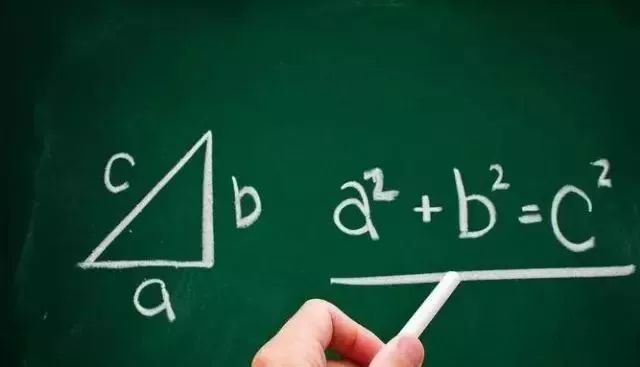1
初等几何

在希腊语中，“几何学”是由“地”与“测量”合并而来的，本来有测量土地的含义，意译就是“测地术”。“几何学”这个名词，系我国明代数学家根据读音译出的，沿用至今。
现在的初等几何主要是指欧几里得几何，它是讨论图形(点、线、面、角、圆等)在运动下的不变性质的科学。例如，欧氏几何中的两点之间的距离，两条直线相交的交角大小，半径是r的某一圆的面积等都是一些运动不变量。
初等几何作为一门课程来讲，安排在初等代数之后；然而在历史上，几何学的发展曾优先于代数学，它主要被认为是古希腊人的贡献。
几何学舍弃了物质所有的其它性质，只保留了空间形式和关系作为自己研究的对象，因此它是抽象的。这种抽象决定了几何的思维方法，就是必须用推理的方法，从一些结论导出另一些新结论。定理是用演绎的方式来证明的，这种论证几何学的代表作，便是公元前三世纪欧几里得的《原本》，它从定义与公理出发，演绎出各种几何定理。
现在中学《平面三角》中关于三角函数的理论是15世纪才发展完善起来的，但是它的一些最基本的概念，却早在古代研究直角三角形时便己形成。因此，可把三角学划在初等几何这一标题下。
古代埃及、巴比伦、中国、希腊都研究过有关球面三角的知识。公元前2世纪，希帕恰斯制作了弦表，可以说是三角的创始人。后来印度人制作了正弦表；阿拉伯的阿尔·巴塔尼用计算sinθ值的方法来解方程，他还与阿布尔·沃法共同导出了正切、余切、正割、余割的概念；赖蒂库斯作了较精确的正弦表，并把三角函数与圆弧联系起来。
由于直角三角形是最简单的直线形，又具有很重要的实用价值，所以各文明古国都极重视它的研究。我国《周髀算经》一开始就记载了周朝初年(约公元前1100年左右)的周公与学者商高的对话，其中就谈到“勾三股四弦五”，即勾股定理的特殊形式；还记载了在周公之后的陈子，曾用勾股定理和相似图形的比例关系，推算过地球与太阳的距离和太阳的直径，同时为勾股定理作的图注达几十种之多。在国外，传统称勾股定理为毕达哥拉斯定理，认为它的第一个一致性的证明源于毕氏学派(公元前6世纪)，虽然巴比伦人在此以前1000多年就发现了这个定理。到现在人们对勾股定理已经至少提供了370种证明。
19世纪以来，人们对于关于三角形和圆的初等综合几何，又进行了深入的研究。至今这一研究领域仍然没有到头，不少资料已引申到四面体及伴随的点、线、面、球。

2
射影几何

射影几何学是一门讨论在把点射影到直线或平面上的时候，图形的不变性质的一门几何学。幻灯片上的点、线，经过幻灯机的照射投影，在银幕上的图画中都有相对应的点线，这样一组图形经过有限次透视以后，变成另一组图形，这在数学上就叫做射影对应。射影几何学在航空、摄影和测量等方面都有广泛的应用。
射影几何是迪沙格和帕斯卡在1639年开辟的。迪沙格发表了—本关于圆维曲线的很有独创性的小册子，从开普勒的连续性原理开始，导出了许多关于对合、调和变程、透射、极轴、极点以及透视的基本原理，这些课题是今天学习射影几何这门课程的人所熟悉的。年仅16岁的帕斯卡得出了一些新的、深奥的定理，并于9年后写了一份内容很丰富的手稿。18世纪后期，蒙日提出了二维平面上的适当投影表达三维对象的方法，因而从提供的数据能快速算出炮兵阵地的位置，避开了冗长的、麻烦的算术运算。
射影几何真正独立的研究是由彭赛勒开创的。1822年，他发表了《论图形的射影性质》一文，给该领域的研究以巨大的推动作用。他的许多概念被斯坦纳进一步发展。1847年，斯陶特发表了《位置几何学》一书，使射影几何最终从测量基础中解脱出来。
后来证明，采用度量适当的射影定义，能在射影几何的范围内研究度量几何学。将一个不变二次曲线添加到平面上的射影几何中，就能得到传统的非欧几何学。在19世纪晚期和20世纪初期，对射影几何学作了多种公设处理，并且有限射影几何也被发现。事实证明，逐渐地增添和改变公设，就能从射影几何过渡到欧几里得几何，其间经历了许多其它重要的几何学。

3
解析几何

解析几何即坐标几何，包括平面解析几何和立体解析几何两部分。解析几何通过平面直角坐标系和空间直角坐标系，建立点与实数对之间的一一对应关系，从而建立起曲线或曲面与方程之间的一一对应关系，因而就能用代数方法研究几何问题，或用几何方法研究代数问题。
在初等数学中，几何与代数是彼此独立的两个分支；在方法上，它们也基本是互不相关的。解析几何的建立，不仅由于在内容上引入了变量的研究而开创了变量数学，而且在方法上也使几何方法与代数方法结合起来。
1637年，笛卡儿发表了《方法论》及其三个附录，他对解析几何的贡献，就在第三个附录《几何学》中，他提出了几种由机械运动生成的新曲线。在《平面和立体轨迹导论》中，费尔马解析地定义了许多新的曲线。在很大程度上，笛卡儿从轨迹开始，然后求它的方程；费尔马则从方程出发，然后来研究轨迹。这正是解析几何基本原则的两个相反的方面，“解析几何”的名称是以后才定下来的。
这门课程达到现在课本中熟悉的形式，是100多年以后的事。象今天这样使用坐标、横坐标、纵坐标这几个术语，是莱布尼兹于1692年提出的。1733年，年仅18岁的克雷洛出版了《关于双重曲率曲线的研究》一书，这是最早的一部空间解析几何著作。1748年，欧拉写的《无穷分析概要》，可以说是符合现代意义的第一部解析几何学教程。1788年，拉格朗日开始研究有向线段的理论。1844年，格拉斯曼提出了多维空间的概念，并引入向量的记号。于是多维解析几何出现了。
解析几何在近代的发展，产生了无穷维解析几何和代数几何等一些分支。普通解析几何只不过是代数几何的一部分，而代数几何的发展同抽象代数有着密切的联系。

4
非欧几何

非欧几何有三种不同的含义：狭义的，单指罗氏(罗巴切夫斯基)几何；广义的，泛指一切和欧氏(欧几里得)几何不同的几何；通常意义的，指罗氏几何和黎曼几何。
欧几里得的第5公设(平行公设)在数学史上占有特殊的地位，它与前4条公设相比，性质显得太复杂了。它在《原本》中第一次应用是在证明第29个定理时，而且此后似乎总是尽量避免使用它。因此人们怀疑第五公设的公理地位，并探索用其它公理来证明它，以使它变为一条定理。在三千多年的时间中，进行这种探索并有案可查的就达两千人以上，其中包括许多知名的数学家，但他们都失败了。
罗巴契夫斯基于1826年，鲍耶于1832年发表了划时代的研究结果，开创了非欧几何。在这种几何中，他们假设“过不在已知直线上的一点，可以引至少两条直线平行于已知直线”，用以代替第五公设，同时保留了欧氏几何的其它公设。
1854年，黎曼推出了另一种非欧几何。在这种几何中，他假设“过已知直线外一点，没有和已知直线平行的直线可引”，用以代替第5公设，同时保留了欧氏几何的其它公设。1871年，克莱因把这3种几何：罗巴契夫斯基—鲍耶的、欧几里得的和黎曼的分别定名为双曲几何、抛物几何和椭圆几何。
非欧几何的发现不仅最终解决了平行公设的问题——平行公设被证明是独立于欧氏几何的其它公设的，而且把几何学从其传统模型中解放出来，创造了许多不同体系的几何的道路被打开了。
1854年，黎曼发表了“关于作为几何学基础的假设的讲演”。他指出：每种不同的(两个无限靠近的点的)距离公式决定了最终产生的空间和几何的性质。1872年，克莱因建立了各种几何系统按照不同变换群不变量的分类方法。
19世纪以后，几何空间概念发展的另一方向，是按照所研究流形的微分几何原则的分类，每一种几何都对应着一种定理系统。1899年，希尔伯特发表了《几何基础》一书，提出了完备的几何公理体系，建立了欧氏几何的严密的基础，并给出了证明一个公理体系的相容性(无矛盾性)、独立性和完备性的普遍原则。按照他的观点，不同的几何空间乃是从属于不同几何公理要求的元素集合。欧氏几何和非欧几何，在大量的几何系统中，只不过是极其特殊的情形罢了。

5
拓扑学

1736年，欧拉发表论文，讨论哥尼斯堡七桥问题。他还提出球面三角形剖分图形顶点、边、面之间关系的欧拉公式，这可以说是拓扑学的开端。
庞加莱于1895～1904年建立了拓扑学，采用代数组合的方法研究拓扑性质。他把欧拉公式推广为欧拉—庞加莱公式，与此有关的理论现在称为同调理论和同伦理论。以后的拓扑学主要按照庞加莱的设想发展。
拓扑学开始是几何学的一个分支，在二十世纪它得到了极大的推广。1906年，弗雷歇发表博士论文，把函数作为一个“点”来看，把函数收敛描绘成点的收敛，这就把康托的点集论和分析学的抽象化联系起来了。他在函数所构成的集合中引入距离的概念，构成距离空间，展开了线性距离空间的理论。在这个基础上，产生了点集拓扑学。在豪斯道夫的《点集论纲要》一书中，出现了更一般的点集拓扑学的完整想法。第二次世界大战后，把分析引进拓扑，发展了微分拓扑。
现在的拓扑学可以粗略地定义为对于连续性的数学研究。任何事物的集合都能在某种意义上构成拓扑空间，拓扑学的概念和理论已基本完组成为数学的基础理论之一，渗入到各个分支，并且成功地应用于电磁学和物理学的研究。

分析学范畴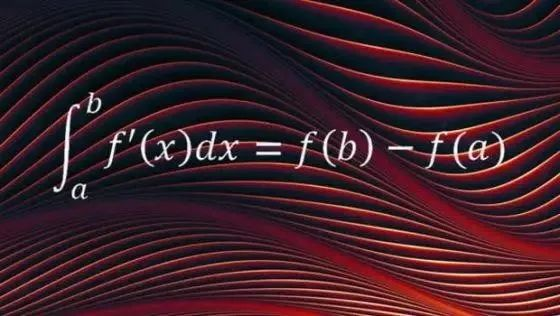1
微积分

微积分学是微分学和积分学的统称，它是研究函数的导数、积分的性质和应用的一门数学分支学科。
微积分的出现具有划时代意义，时至今日，它不仅成了学习高等数学各个分支必不可少的基础，而且是学习近代任何一门自然科学和工程技术的必备工具。现在的微积分学的教程，通常的讲授次序是先极限、再微分、后积分，这与历史顺序正好相反。
在微积分历史中，最初的问题是涉及计算面积、体积和弧长的。阿基米得(公元前3世纪)的方法最接近于现行的积分法。在17世纪探索微积分的至少有十几位大数学家和几十位小数学家。牛顿和莱布尼茨分别进行了创造性的工作，各自独立地跑完了“微积分这场接力赛的最后一棒”。
1609年，开普勒为了计算行星运动第二定律中包含的面积，和在他的论文中讨论的酒桶的体积，而借助了某种积分方法。1635年，卡瓦列利发表了一篇阐述不可分元法的论文，提出卡瓦列利原理，它是计算面积和体积的有价值的工具。1650年，沃利斯把卡瓦列利的方法系统化，并作了推广。
微分起源于作曲线的切线和求函数的极大值或极小值问题。虽然可以追溯到古希腊，但是第一个真正值得注意的先驱工作，是费尔马1629年陈述的概念。1669年，巴罗对微分理论作出了重要的贡献，他用了微分三角形，很接近现代微分法。一般认为，他是充分地认识到微分法为积分法的逆运算的第一个人。
至此，还有什么要做的呢？首要的是，创造一般的符号和一整套形式的解析规则，形成可以应用的微积分学，这项工作是由牛顿和莱布尼兹彼此独立地做出的。接着的工作是在可接受的严格的基础上，重新推导基本理论，这必须等到此课题想到多方面应用之后。柯西和他的后继者们完成了这一工作。
牛顿早在1665年才23岁时，就创造了流数法(微分学)，并发展到能求曲线上任意一点的切线和曲率半径。他的《流数法》写于1671年，但直到死后9年的1736年才发表。牛顿考虑了两种类型的问题，等价于现在的微分和解微分方程。他定义了流数(导数)、极大值、极小值、曲线的切线、曲率、拐点、凸性和凹性，并把它的理论应用于许多求积问题和曲线的求长问题。
牛顿创立的微积分原理是同他的力学研究分不开的，他借此发现、并研究了力学三大定律和万有引力定律，1687年出版了名著《自然哲学的数学原理》。这本书是研究天体力学的，包括了微积分的一些基本概念和原理。
莱布尼茨是在1673年到1676年之间，从几何学观点上独立发现微积分的。1676年，他第一次用长写字母∫表示积分符号，象今天这样写微分和微商。1684年～1686年，他发表了一系列微积分著作，力图找到普遍的方法来解决问题。今天课本中的许多微分的基本原则就是他推导出来的，如求两个函数乘积的n阶导数的法则，现在仍称作菜布尼兹法则。莱布尼兹的另一最大功绩是创造了反映事物本质的数字符号，数学分析中的基本概念的记号，例如微分dx，二级微分dx?，积分∫ydx，导数dy/dx等都是他提出来的，并且沿用至今，非常方便。
牛顿与莱布尼茨的创造性工作有很大的不同。主要差别是牛顿把x和y的无穷小增量作为求导数的手段，当增量越来越小的时候，导数实际上就是增量比的极限，而莱布尼兹却直接用x和y的无穷小增量(就是微分)求出它们之间的关系。
这个差别反映了他们研究方向的不同，在牛顿的物理学方向中，速度之类是中心概念；而在莱布尼兹的几何学方向中，却着眼于面积体积的计算。其它差别是，牛顿自由地用级数表示函数，采用经验的、具体和谨慎的工作方式，认为用什么记号无关紧要；而莱布尼兹则宁愿用有限的形式来表示函数，采用富于想象的、喜欢推广的、大胆的工作方式，花费很多时间来选择富有提示性的符号。
到1700年，现在大学且学习的大部分微积分内容已经建立起来。第一部微积分课本出版于1696年，是洛比达写的。1769年，欧拉论述了二重积分。1773年，拉格朗日考察了三重积分。1837年，波尔查诺给出了级数的现代定义。19世纪分析学的严谨化，是由柯西奠基的。现在课本中的极限、连续性定义、把导数看作差商的极限、把定积分看做和的权限等等，实质上都是柯西给出的。进一步完成这一工作的是威尔斯特拉斯，他给出了现在使用的精确的极限定义，并同狄德金、康托于19世纪70年代建立了严格的实数理论，使微积分有了坚固可靠的逻辑基础。

2
微分方程

凡是表示未知函数和未知函数的导数以及自变量之间的关系的方程，就叫做微分方程。如果未知函数是一元函数，则称为常微分方程，如果未知函数是多元函数，则称为偏微分方积。微分方程的基本问题是在一定条件下，从所给出的微分方程解出未知函数。
微分方程几乎是与微积分同时发展起来的，由于它与力学、物理学的渊源很深，所以在13世纪便已自成一门独立的学科了。两个多世纪来，这一学科已发展得相当完善。
1676年，莱布尼兹在致牛顿的信中，首先提出了“微分方程”这个名称。在他们两人的著作中，都包含了许多微分方程的实例。早期的研究侧重于探讨各类一阶方程的解法，并由此导致了方程的分类。18世纪，欧拉解决了全微分方程和“欧拉方程”(一类高阶变系数线性微分方程)，提出了通解和特解的概念，指出了n阶线性方程通解的结构。其后，泰勒得到了方程的奇解；拉格朗日推导了非齐次线性方程的常数交易法。
对于微分方程组的研究，始于达朗贝尔。19世纪前半叶，柯西开始研究解的存在性和唯一性。19世纪后半叶，数学家们开始利用群论来研究微分方程，由此建立连续群和李群的新理论。庞加莱引入了极限环的概念，李雅普诺夫引入了微分方程组解的稳定性概念。他们的方法都不必直接求解，称为定性理论。1927年，毕尔霍夫建立了“动力系统”的一段定性理论。
一阶偏微分方程的研究首先是从几何学问题开始的。拉格朗日指出，解一阶线性偏微分方程的技巧，在于把它们化为常微分方程。一阶非线性偏微分方程的研究，始于欧拉和拉格朗日，蒙日为偏微分方程的几何理论奠定了基础。到18世纪末叶，在引入奇解、通解、全积分、通积分、特积分等概念之后，偏微分方程已形成一门独立的学科。
二阶偏微分方程的研究，始于18世纪的弦振动理论。通常见的二阶偏微分方程均来自物理或力学的实际问题，它们构成了这门学科中一个独立的系统—数学物理方程。
积分方程源于阿贝尔1826年的工作，但是直到1888年杜·波阿·雷蒙的著作中，才正式提出了积分方程这个名词。1896年开始，伏特拉给出了两类积分方程的一般理论；不久，弗雷德荷姆大体上完成了一类重要的线性积分方程理论。由于这类积分方程常出现在一些物理问题中，因此积分方程论常被包含在数学物理方程内。
现代科学技术，如空间技术、现代物理学、力学等，都有许多问题需要用微分方程来求解，甚至在化学、生物学、医药学、经济学等方面，微分方程的应用也越来越多。

3
微分几何

微分几何这门分支学科主要研究三维欧氏空间中曲线和曲面的内在性质，所谓内在性质就是同几何对象在空间中的位置无关的性质。它以微积分、微分方程这些分支学科的理论为研究工具。或简单地说，微分几何就是用分析方法研究几何性质。
微分几何的发端可见于1731年克莱洛的著作中。蒙日1809年的著作包含了这一学科的雏型；欧拉研究了曲面的一般理论；高斯1827年的《关于曲面的一般研究》一书，论述了曲面理论，创立了内蕴几何学，奠定了曲面微分几何的基础。1887～1896年，达布的《曲面一般理论的讲义》集曲线和曲面微分几何之大成。
变换理论对于微分几何的影响，产生了射影微分几何、仿射微分几何等分支。二十世纪初，出现了对非充分光滑曲线和曲面以及曲线曲面的整体问题的研究，形成现代微分几何。1923年，嘉当提出了一般联络的理论。1945年，陈省身建立了代数拓扑和微分几何的联系，他又是纤维丛概念的创建人之一。

4
函数论

函数论包括复变函数论和实变函数论，但有时也单指复变函数论(或复分析)而言。
复数概念出现于16世纪，但对它的全面掌握和广泛运用，却迟至18世纪。自变量是复数的函数，叫做复变函数。如果复变函数在某一区域内除了可能有有限个例外点之外，处处有导数，那么这个伏辩函数叫做在这个区域内的解析函数；例外点叫做奇点。复变函数论主要研究解析函数的性质。
复变函数的研究是从18世纪开始的。30～40年代，欧拉利用幂级数详细讨论了初等复变函数的性质。达朗贝尔于1752年得出复变函数可微的必要条件(即“柯西—黎曼条件”)。拉普拉斯也考虑过复变函数的积分。
复变函数的全面发展是在19世纪。1825年，柯西讨论了虚限定积分，1831年他实质上推出了柯西积分公式，并在此基础上建立了一整套复变函数微分和积分的理论。黎曼1851年的博士论文《复变函数论的基础》，奠定了复变函数论的基础。他推广了单位解析函数到多位解析函数；引入了“黎曼曲面”的重要概念，确立了复变因数的几何理论基础；证明了保角映射基本定理。威尔斯特拉斯完全摆脱了几何直观，以幂级数为工具，用严密的纯解析推理展开了函数论。定义解析函数是可以展开为幂级数的函数，围绕着奇点研究函数的性质。近几十年来，复变函数论又有很大的推进。
复变函数论是解决工程技术问题的有力工具，飞机飞行理论、热运动理论、流体力学理论、电场和弹性理论等中的很多问题。
实变函数的发展较晚，其中积分论是它的重要组成部分。容度和测度是线段长度概念的推广，是为了推广积分的概念而建立起来的。1893年，约当给出了“约当容度”的概念，并用于讨论积分。1894年，斯提捷首先推广了积分概念，得到了“斯提捷积分”。1898年，波莱尔改进了容度的概念，他称之为‘测度”。下一步决定性的进展是1902年勒贝格改进了测度理论，建立了“勒贝格测度”、“勒贝格积分”等概念。1904年，他完全解决了黎曼可积性的问题。后来，数学家们对积分的概念又作了种种推广和探索。
实变函数的另一个领域是函数构造论。1885年，威尔斯特拉斯证明：连续函数必可表示为一致收敛的多项式级数。这一结果和切比雪夫斯基最佳逼近论，是函数构造论的开端。近年来，这个方向的研究十分活跃。

5
泛函分析

本世纪初，出现了一个广阔的新领域——泛函分析，它是古典分析观点的推广。近几十年来，由于分析学中许多新分支的形成，从而发现在代数、几何、分析中不同领域之间的某些方面的类似。其次，几何与集合论的结合产生了抽象空间的理论，将函数看成函数空间中的点。再加上实变函数论以及近世代数的感念和方法的影响，就产生了泛函分析。它综合函数论，几何和代数的观点，研究无穷维向量空间上的函数、算子和极限理论。
19世纪末，弗尔太拉和二十世纪初阿达玛的著作中已出现泛函分析的萌芽。随后希尔伯特、海令哲开创了“希尔伯将空间”的研究，黎斯、冯·诺伊曼等人在这方面都有重要的建树。

展开全文• 《线性代数几何意义》之四_(_向量组及向量空间的几何意义_).pdf
• 我们根据在Dynkin图（与相关的Borcherds超代数的图一致）中编码的生成器和关系，进一步发展了最近提出的张量层次代数的定义。 我们将其应用于将灰色节点添加到秩r + 1 Kac-Moody代数g $$\ mathfrak {g}$$ +的...
• 第一章 什么是线性代数 代数的功能是把许多看似不相关的事物进行抽象，以提高效率，把许多看似不相关的问题化归为一类问题。 1.1 线性的几何意义 一元线性函数的概念 定义：f(x)=kxf(x) = kxf(x)=kx（注：f(x)=ax+...
第一章 什么是线性代数

代数的功能是把许多看似不相关的事物进行抽象，以提高效率，把许多看似不相关的问题化归为一类问题。

1.1 线性的几何意义
一元线性函数的概念
定义：

f

(

x

)

=

k

x

f(x) = kx

（注：

f

(

x

)

=

a

x

+

b

f(x) = ax+b

在这里不再称为线性函数）。几何意义：直线。代数意义：
可加性：若

f

(

x

)

f(x)

是线性的，则有

f

(

x

1

+

x

2

)

=

f

(

x

1

)

+

f

(

x

2

)

f(x_1+x_2) = f(x_1)+f(x_2)

。说明：所有起因的累加所导致的结果完全等于每个起因独自所引起的结果的累加；比例性（齐次性/数乘性/均匀性）：若

f

(

x

)

f(x)

是线性的，则有

f

(

k

x

)

=

k

f

(

x

)

f(kx) = kf(x)

。说明：没有输入信号时输出也为零，有几倍的输入量刚好就有几倍的输出量，增量是倍数关系，存量也是倍数关系；以上两条性质合并起来：

f

(

k

x

1

+

k

x

2

)

=

k

f

(

x

1

)

+

k

f

(

x

2

)

f(kx_1+kx_2) = kf(x_1)+kf(x_2)

，即：线性组合的函数，等于函数的线性组合。
多元线性函数的几何意义：从一元线性函数如何推广到

n

n

元线性函数
将

f

(

x

1

)

=

k

1

x

1

f(x_1) = k_1x_1

扩展到

f

(

x

1

,

x

2

)

=

k

1

x

1

+

k

2

x

2

f(x_1,x_2) = k_1x_1+k_2x_2

从

f

(

x

1

)

=

k

1

x

1

f(x_1) = k_1 x_1

到

f

(

x

1

,

x

2

)

=

k

1

x

1

f(x_1,x_2) = k_1x_1

：二维平面坐标系

x

1

∼

f

(

x

1

)

x_1\sim f(x_1)

里有一根直线图形

f

(

x

1

)

=

k

1

x

1

f(x_1) = k_1 x_1

，从原点以垂直于原坐标系“生长”出坐标轴

x

2

x_2

。原直线

f

(

x

1

)

=

k

1

x

1

f(x_1) = k_1x_1

沿着坐标轴

x

2

x_2

滑动，无数个平行的直线被

x

2

x_2

轴“串”起来，平铺得到平面

f

(

x

1

,

x

2

)

=

k

1

x

1

f(x_1,x_2) = k_1 x_1

，其是由无数的直线铺成的，因此，平面也是“线性”的。从

f

(

x

1

,

x

2

)

=

k

1

x

1

f(x_1,x_2) = k_1 x_1

到

f

(

x

1

,

x

2

)

=

k

1

x

1

+

k

2

x

2

f(x_1,x_2) = k_1 x_1+k_2 x_2

：如图所示，将

f

(

x

1

,

x

2

)

=

k

1

x

1

f(x_1,x_2) = k_1 x_1

（白色）与

f

(

x

1

,

x

2

)

=

k

2

x

2

f(x_1,x_2) = k_2 x_2

（白色）叠加，得到一个仍过原点

O

O

的平面（彩色）。图1.1.1 彩色平面为叠加后的平面

将

f

(

x

1

,

x

2

)

=

k

1

x

1

+

k

2

x

2

f(x_1,x_2) = k_1 x_1+k_2 x_2

继续扩展到

f

(

x

1

,

x

2

,

⋯

,

x

n

)

=

k

1

x

1

+

k

2

x

2

+

⋯

+

k

n

x

n

f(x_1,x_2,\cdots,x_n) = k_1 x_1+k_2 x_2+\cdots +k_n x_n

可以想象，由二元线性函数

f

(

x

1

,

x

2

)

=

k

1

x

1

+

k

2

x

2

f(x_1,x_2) = k_1 x_1+k_2 x_2

继续扩展到三元及

n

n

元的线性函数

f

(

x

1

,

x

2

,

⋯

,

x

n

)

=

k

1

x

1

+

k

2

x

2

+

⋯

+

k

n

x

n

f(x_1,x_2,\cdots,x_n) = k_1 x_1+k_2 x_2+\cdots +k_n x_n

（坐标系扩展到四维及

n

n

维）后，其几何图形是一个扩展意义上的“平面”，称为超平面。
1.2 线性映射和线性变换的几何意义
线性映射
定义

T

:

x

→

y

,

x

↦

k

x

T: \boldsymbol{x} \rightarrow \boldsymbol{y}, \boldsymbol{x} \mapsto k \boldsymbol{x}

表示从自变量数的集合

x

\boldsymbol x

到因变量数的集合

y

\boldsymbol y

的映射（一个动作），其中

T

T

称为线性算子。
几何意义
一维线性函数式的情形图1.2.1 集合映射

图中给出了一元线性齐次函数

f

(

x

)

=

k

x

f (x) = kx

当

k

k

取不同的数时的映射对应关系。在三个图中，有一 个共性就是元素

0

0

必然映射到元素

0

0

，这是线性映射的最基本要求。
对于一元线性齐次函数

f

(

x

)

=

k

x

f (x) = kx

，集合

x

\boldsymbol x

和集合

y

\boldsymbol y

都是实数，因此可以分别用一坐标轴表示。这样，我们就可以用坐标轴上点之间的映射，来替代图1.2.1的映射。图1.2.2-1 坐标轴映射 (1)图1.2.2-2 坐标轴映射 (2)

如果把两个坐标轴的原点重合（因为

0

0

必然映射到

0

0

），再把两个坐标轴的夹角调整到

π

2

\dfrac \pi 2

，就得到了笛卡尔坐标系（

k

>

0

k>0

的情况），如图1.2.2-2左所示。
图中

a

a

、

b

b

分别映射到

a

’

a’

、

b

’

b’

，如果把点

a

a

、

a

′

a'

、

b

b

和

b

′

b'

分别与原点

O

O

连起来，就会得到线段

O

a

Oa

、

O

b

Ob

、

O

a

’

Oa’

、

O

b

′

Ob'

。也就是说：线性映射就是把线段映射到线段。也可以说：线性映射就是把向量映射成向量。
同样，线性映射也满足线性的可加性和比例性的性质（如图1.2.2-2右）：
可加性：

x

x

轴上的两向量的和，映射到

y

y

轴得到两个

x

x

轴向量分别映射得到的

y

y

轴向量的和，表示为

T

(

α

⃗

+

β

⃗

)

=

T

α

⃗

+

T

β

⃗

T(\vec \alpha + \vec \beta) = T\vec \alpha + T \vec \beta

；比例性：

x

x

轴向量的倍数映射到的

y

y

轴向量等于

x

x

轴向量映射的

y

y

轴向量的倍数，表示为

T

(

k

α

⃗

)

=

k

T

α

⃗

T(k\vec \alpha) = kT\vec \alpha

；
其中，

T

:

x

→

y

,

x

↦

k

x

T: \boldsymbol{x} \rightarrow \boldsymbol{y}, \boldsymbol{x} \mapsto k \boldsymbol{x}

。
二维线性函数式的情形
考察二维线性函数式：

y

⃗

=

K

x

⃗

⇔

(

y

1

y

2

)

=

[

a

1

a

2

b

1

b

2

]

(

x

1

x

2

)

\vec y = \boldsymbol K \vec x \quad \Leftrightarrow \quad \left(\begin{array}{l} {y_{1}} \\ {y_{2}} \end{array}\right)=\left[\begin{array}{ll} {a_{1}} & {a_{2}} \\ {b_{1}} & {b_{2}} \end{array}\right]\left(\begin{array}{l} {x_{1}} \\ {x_{2}} \end{array}\right)

因为向量

x

⃗

\vec x

和

y

⃗

\vec y

都是二维向量，所有任意的向量

x

⃗

\vec x

的集合将构成平面

π

1

\pi_1

，所有任意的向量

y

⃗

\vec y

的集合将构成平面

π

2

\pi_2

，并在两个平面上分别构建平面直角坐标系。因而，二维线性函数就构成了两个二维平面之间由矩阵

K

=

[

a

1

a

2

b

1

b

2

]

\boldsymbol{K}=\left[\begin{array}{ll} {a_{1}} & {a_{2}} \\ {b_{1}} & {b_{2}} \end{array}\right]

所确定的映射关系。图1.2.3

将两个平面的原点重合并垂直相交，得到三维空间。例如，矩阵

K

\boldsymbol K

将

π

1

\pi_1

平面上的向量

α

⃗

1

\vec \alpha_1

映射为

π

2

\pi_2

平面上的向量

β

⃗

1

\vec \beta_1

，这也同样满足可加性、比例性（如图1.2.3右）和线性可加性（如图1.2.4）图1.2.4 三维空间映射-线性可加性

不难发现，由于平行四边形法则，一个平面上的平行四边形，映射到另一平面后变为另一平行四边形（他们可能全等、相似或既不全等也不相似）。同样也可能由一个圆，映射为圆、椭圆或者一根线段，特别情况下被映射为原点（如图1.2.5）。图1.2.5 三维空间映射-圆映射为椭圆

线性变换
若数域

F

F

上线性空间

V

V

中的变换

T

T

若满足条件

T

(

α

⃗

+

β

⃗

)

=

T

α

⃗

+

T

β

⃗

(

α

⃗

,

β

⃗

∈

V

)

T

(

k

α

⃗

)

=

k

T

α

⃗

(

k

∈

F

,

α

⃗

∈

V

)

T(\vec \alpha + \vec \beta) = T\vec \alpha + T \vec \beta \quad(\vec \alpha , \vec \beta \in V)\\ T(k \vec \alpha) = kT\vec \alpha \quad(k \in F , \vec \alpha \in V)

则称为

V

V

中的线性变换。也就是说，如果映射是发生在一个集合上的同一个坐标系中，线性映射就被称为线性变换。图1.2.6 线性变换

例如，在二维向量空间中，把所有向量绕原点作同样角度的旋转是一个线性变换（旋转变换），在三维向量空间中，把每一个向量投影在坐标面

x

O

y

xOy

上，也是一个线性变换（投影变换），不难发现，它们都符合可加性和比例性。

第二章 向量的基本几何意义
2.1 向量概念的几何意义
自由向量
一个确定的向量不允许改变方向。在一条直线上平移的向量称为自由向量（滑动向量）。大小相等、方向相同的两个向量相等。因此，一个向量在保持长度和方向不变的条件下可以自由平移。
向量的数学表示

n

n

维空间向量以

n

n

维向量空间中的原点作为起点。用有序数组表示，或分解为

n

n

个单位坐标向量的线性表示。向量没有除法。
2.3 向量的内积（点积，数量积）
定义：

a

⃗

⋅

b

⃗

=

a

b

cos

⁡

θ

a

⃗

⋅

b

⃗

=

a

x

b

x

+

a

y

b

y

+

a

z

b

z

\vec a \cdot \vec b = ab\cos \theta\\ \vec a \cdot \vec b = a_xb_x+a_yb_y+a_zb_z

几何意义：一个向量在另一个向量上的投影的积；内积值越大，两个向量的在方向上就越接近；内积值 越小，两个向量的在方向上就越相反；内积值为0，两向量垂直。  一个重要结论：若

a

⃗

\vec a

为某个坐标轴的单位坐标向量，则

a

⃗

⋅

b

⃗

\vec a \cdot \vec b

就是就是

b

⃗

\vec b

在此坐标轴上的坐标值 。  正交变换：
定理1：设

σ

\sigma

是

V

V

的一个变换。若

∀

a

⃗

,

b

⃗

\forall \vec a, \vec b

都有

∣

σ

(

a

⃗

)

+

σ

(

b

⃗

)

∣

=

∣

a

⃗

+

b

⃗

∣

\left| \sigma(\vec a)+\sigma(\vec b) \right| = \left| \vec a+\vec b\right|

则

σ

\sigma

是

V

V

的正交变换。其几何意义是“保持以

V

V

中任意两个向量为邻边的平行四边形的对角线之长不变” 。  定理2：设

σ

\sigma

是

V

V

的一个变换。若

σ

\sigma

既是保长度变换又是保夹角变换，则

σ

\sigma

必为正交变换。
2.4 向量的外积（叉积，向量积）
定义：

a

⃗

×

b

⃗

=

(

a

y

b

z

−

a

z

b

y

,

a

z

b

x

−

a

x

b

z

,

a

x

b

y

−

a

y

b

x

)

\vec a \times \vec b = \left(a_{y} b_{z}-a_{z} b_{y}, \ a_{z} b_{x}-a_{x} b_{z},\ a_{x} b_{y}-a_{y} b_{x}\right)

；

a

⃗

×

b

⃗

=

∣

a

⃗

∣

∣

b

⃗

∣

sin

⁡

θ

e

^

n

\vec a \times \vec b = \left| \vec a \right| \left| \vec b \right| \sin \theta \hat e_{\rm n}

。 几何意义：叉积的模长等于

a

⃗

,

b

⃗

\vec a, \vec b

构成的平行四边形的面积。物理意义：如

v

⃗

=

ω

⃗

×

r

⃗

\vec v = \vec \omega \times \vec r

。

a

⃗

×

c

⃗

0

\vec a \times \vec c_0

的几何意义（

c

⃗

0

\vec c_0

为单位向量）：图2.4.1
的几何意义

2.5 向量混合运算的几何意义
向量叉积的分配律

(

a

⃗

+

b

⃗

)

×

c

⃗

=

a

⃗

×

c

⃗

+

b

⃗

×

c

⃗

\left( \vec a +\vec b \right) \times \vec c = \vec a \times \vec c + \vec b \times \vec c

的几何解释
解释一（“一投一转，再加一伸”）：

a

⃗

\vec a

、

b

⃗

\vec b

、

a

⃗

+

b

⃗

\vec a + \vec b

分别按照[此方法](#2.4 向量的外积（向量积）)作出

a

⃗

′

\vec a'

、

b

⃗

′

\vec b'

、

a

⃗

′

+

b

⃗

’

\vec a' + \vec b’

以及

a

⃗

×

c

⃗

0

\vec a\times \vec c_0

、

b

⃗

×

c

⃗

0

\vec b\times \vec c_0

、

(

a

⃗

+

b

⃗

)

×

c

⃗

0

\left(\vec a + \vec b\right) \times \vec c_0

，显然，这三组向量都可构成平行四边形，即

a

⃗

×

c

⃗

0

+

b

⃗

×

c

⃗

0

=

(

a

⃗

+

b

⃗

)

×

c

⃗

0

\vec a\times \vec c_0+\vec b\times \vec c_0=\left(\vec a + \vec b\right) \times \vec c_0

再将单位向量

c

⃗

0

\vec c_0

伸缩为一般的向量

c

⃗

=

∣

c

⃗

∣

c

⃗

0

\vec c = \left| \vec c\right| \vec c_0

，即等式两边同乘常数

∣

c

⃗

∣

\left| \vec c\right|

，得：

a

⃗

×

c

⃗

0

∣

c

⃗

∣

+

b

⃗

×

c

⃗

0

∣

c

⃗

∣

=

(

a

⃗

+

b

⃗

)

×

c

⃗

0

∣

c

⃗

∣

a

⃗

×

c

⃗

+

b

⃗

×

c

⃗

=

(

a

⃗

+

b

⃗

)

×

c

⃗

(

a

⃗

+

b

⃗

)

×

c

⃗

=

a

⃗

×

c

⃗

+

b

⃗

×

c

⃗

\begin{aligned} \vec a\times \vec c_0\left| \vec c\right|+\vec b\times \vec c_0\left| \vec c\right| &= \left(\vec a + \vec b\right) \times \vec c_0\left| \vec c\right|\\ \vec a\times \vec c+\vec b\times \vec c &= \left(\vec a + \vec b\right) \times \vec c\\ \left( \vec a +\vec b \right) \times \vec c &= \vec a \times \vec c + \vec b \times \vec c \end{aligned}图2.5.1 向量叉积的分配律的几何解释1

解释二（面向量）：面向量的加法运算同样满足平行四边形法则和三角形法则，具体过程略。图2.5.2 向量叉积的分配律的几何解释2

向量混合积

(

a

⃗

×

b

⃗

)

⋅

c

⃗

\left(\vec a \times \vec b \right) \cdot \vec c

的几何解释

(

a

⃗

×

b

⃗

)

⋅

c

⃗

=

(

b

⃗

×

c

⃗

)

⋅

a

⃗

=

(

c

⃗

×

a

⃗

)

⋅

b

⃗

\left(\vec a \times \vec b \right) \cdot \vec c = \left(\vec b \times \vec c \right) \cdot \vec a = \left(\vec c \times \vec a \right) \cdot \vec b

，其绝对值表示以这三个向量为棱的平行六面体的体积。如果它们顺序组成右手系，那么积符号为正，反之为负。图2.5.3 向量混合积的几何解释

2.6 向量的积和张量之间的关系

a

⃗

,

b

⃗

\vec{a}, \vec{b}

的张量积：

a

⃗

b

⃗

=

a

x

b

x

i

⃗

i

⃗

+

a

y

b

y

j

⃗

j

⃗

+

(

a

x

b

y

i

⃗

j

⃗

+

a

y

b

x

j

⃗

i

⃗

)

\vec{a} \vec{b}=a_{x} b_{x} \vec{i} \vec{i}+a_{y} b_{y} \vec{j} \vec{j}+\left(a_{x} b_{y} \vec{i} \vec{j}+a_{y} b_{x} \vec{j} \vec{i}\right)

，其第一部分包含了

a

⃗

\vec a

和

b

⃗

\vec b

内积的结果，第二部分包含了

a

⃗

\vec a

和

b

⃗

\vec b

外积或行列式的结果，即：

a

⃗

b

⃗

=

(

a

⃗

⋅

b

⃗

)

+

(

a

⃗

×

b

⃗

)

\vec{a} \vec{b}=\left(\vec{a} \cdot \vec{b}\right)+\left(\vec{a} \times \vec{b}\right)

2.7 向量有没有除法
点积的除法和叉积的除法图2.7.1 点积的除法和叉积的除法的几何解释

假设

a

⃗

⋅

b

⃗

=

c

\vec a \cdot \vec b = c

和

a

⃗

×

b

⃗

=

c

⃗

\vec a \times \vec b = \vec c

，且已知

a

⃗

,

c

,

c

⃗

\vec a,\ c,\ \vec c

，求

b

⃗

\vec b

。根据上图可以发现，

b

⃗

,

b

⃗

’

,

b

⃗

’

’

,

b

⃗

’

’

′

\vec b,\ \vec b’,\ \vec b’’,\ \vec b’’'

都满足等式，故点积和叉积均没有除法。
通过联立求解除法的表达式
解方程

{

a

⃗

⋅

b

⃗

=

c

a

⃗

×

b

⃗

=

c

⃗

\left\{\begin{array}{l} \vec{a} \cdot \vec{b}=c \\ \vec{a} \times \vec{b}=\vec{c} \end{array}\right.

可解得向量除法的表达式

b

⃗

=

c

a

⃗

−

a

⃗

×

c

⃗

a

⃗

⋅

a

⃗

\vec{b}=\frac{c \vec{a}-\vec{a} \times \vec{c}}{\vec{a} \cdot \vec{a}}

2.8 向量的投影和的几何解释
多个或有限个向量的和在任意轴上投影等于各个向量在同一轴上投影的和。图2.8.1 多个向量在任意轴上的投影和的几何解释

多个或有限个向量的和在任意平面上投影等于各个向量在同一平面上投影的和。图2.8.2 多个向量在任意平面上的投影和的几何解释

2.9 变向量的几何意义
二维变向量的几何图形
在二维平面上，二维向量

(

x

1

,

x

2

)

(x_1,x_2)

的两个分量全部为可变量，称之为二维变向量。若

x

1

,

x

2

x_1,x_2

取遍所有实数，则其表示整个二维平面。若：
固定

x

1

x_1

（或

x

2

x_2

）为

a

a

，则所有向量的末端在一条竖直（或水平）的直线上，因而表示一条直线；  向量的分量之间有线性关系，如

x

2

=

a

x

1

+

b

x_2 = ax_1+b

，则所有向量的末端在直线

x

2

=

a

x

1

+

b

x_2 = ax_1+b

上，因而表示直线

x

2

=

a

x

1

+

b

x_2 = ax_1+b

。图2.9.1 二维变向量的分量之间有线性关系

(

x

1

,

a

x

1

+

b

)

\left(x_1, ax_1+b\right)

可表示为

(

x

1

,

a

x

1

+

b

)

=

(

x

1

,

a

x

1

)

+

(

0

,

b

)

\left(x_1, ax_1+b\right)=(x_1,ax_1)+(0,b)

，几何解释为：直线

(

x

1

,

a

x

1

+

b

)

\left(x_1, ax_1+b\right)

是由直线

(

x

1

,

a

x

1

)

(x_1,ax_1)

沿向量

(

0

,

b

)

(0,b)

平移

b

b

得到的。图2.9.2
的几何解释

三维变向量的几何图形
若

x

1

,

x

2

,

x

3

x_1,x_2,x_3

取遍所有实数，则其表示整个三维空间。若：
当

(

x

1

,

x

2

,

x

3

)

(x_1,x_2,x_3)

中一个变量取为定值时，分别表示垂直于三个坐标轴的平面；  当

(

x

1

,

x

2

,

x

3

)

(x_1,x_2,x_3)

中两个变量取为定值时，如

(

a

1

,

a

2

,

x

3

)

(a_1,a_2,x_3)

表示平行于

x

3

x_3

轴的直线

{

x

1

=

a

1

x

2

=

a

2

\left\{\begin{array}{l} x_{1}=a_{1} \\ x_{2}=a_{2} \end{array}\right.

；图2.9.3
的几何图形

当有线性关系时，如

(

x

1

,

x

2

,

a

x

1

+

b

x

2

)

(x_1,x_2,ax_1+bx_2)

可进行向量分解，表示为

(

x

1

,

x

2

,

a

x

1

+

b

x

2

)

=

(

x

1

,

0

,

a

x

1

)

+

(

0

,

x

2

,

b

x

2

)

=

x

1

(

1

,

0

,

a

)

+

x

2

(

0

,

1

,

b

)

\begin{aligned} (x_1,x_2,ax_1+bx_2)&=\left(x_{1}, 0, a x_{1}\right)+\left(0, x_{2}, b x_{2}\right)\\ &=x_{1}(1,0, a)+x_{2}(0,1, b) \end{aligned}

当

x

1

,

x

2

x_1,x_2

独立取遍所有实数时，

x

1

(

1

,

0

,

a

)

+

x

2

(

0

,

1

,

b

)

x_{1}(1,0, a)+x_{2}(0,1, b)

所形成的无数个向量覆盖了一个平面。实际上，这是一个被常向量

(

1

,

0

,

a

)

(1,0,a)

和

(

0

,

1

,

b

)

(0,1,b)

所张成的向量空间，记作

s

p

a

n

{

(

1

,

0

,

a

)

,

(

0

,

1

,

b

)

}

{\rm span}\left\{ (1,0,a),(0,1,b)\right\}

。因此有以下等式：

(

x

1

,

x

2

,

a

x

1

+

b

x

2

)

=

s

p

a

n

{

(

1

,

0

,

a

)

,

(

0

,

1

,

b

)

}

(x_1,x_2,ax_1+bx_2) = {\rm span}\left\{ (1,0,a),(0,1,b)\right\}

类似地

(

x

1

,

x

2

,

a

x

1

+

b

x

2

+

c

)

=

(

x

1

,

x

2

,

a

x

1

+

b

x

2

)

+

(

0

,

0

,

c

)

(x_1,x_2,ax_1+bx_2+c) = (x_1,x_2,ax_1+bx_2)+(0,0,c)

同样表示

(

x

1

,

x

2

,

a

x

1

+

b

x

2

)

(x_1,x_2,ax_1+bx_2)

的平面沿着向量

(

0

,

0

,

c

)

(0,0,c)

平移了

c

c

。
变向量的应用
二阶线性方程组

{

x

2

=

a

1

x

1

+

b

1

x

2

=

a

2

x

1

+

b

2

\left\{\begin{array}{l} x_{2}=a_{1} x_{1}+b_{1} \\ x_{2}=a_{2} x_{1}+b_{2} \end{array}\right.

可以用变向量的交

(

x

1

,

a

1

x

1

+

b

1

)

⋂

(

x

1

,

a

2

x

1

+

b

2

)

\left(x_{1}, a_{1} x_{1}+b_{1}\right) \bigcap\left(x_{1}, a_{2} x_{1}+b_{2}\right)

来等价表示。可以想象，两个变向量相同的向量就是他们的交集。如果两个向量相同，那么向量的夹角就会为零，根据行列式的几何意义知道，此时行列式为零，即

∣

x

1

a

1

x

1

+

b

1

x

1

a

2

x

1

+

b

2

∣

=

0

\left|\begin{array}{ll} x_{1} & a_{1} x_{1}+b_{1} \\ x_{1} & a_{2} x_{1}+b_{2} \end{array}\right|=0

化简得到

x

1

=

0

x_1 = 0

或

x

1

=

b

1

−

b

2

a

2

−

a

1

x_1 = \dfrac{b_1-b_2}{a_2-a_1}

，进而得到两对线性相关的向量：

(

0

,

b

1

)

,

(

0

,

b

2

)

(0,b_1),\ (0,b_2)

和

(

b

1

−

b

2

a

2

−

a

1

,

a

2

b

1

−

a

1

b

2

a

2

−

a

1

)

,

(

b

1

−

b

2

a

2

−

a

1

,

a

2

b

1

−

a

1

b

2

a

2

−

a

1

)

\left(\dfrac{b_{1}-b_{2}}{a_{2}-a_{1}}, \dfrac{a_{2} b_{1}-a_{1} b_{2}}{a_{2}-a_{1}}\right),\ \left(\dfrac{b_{1}-b_{2}}{a_{2}-a_{1}}, \dfrac{a_{2} b_{1}-a_{1} b_{2}}{a_{2}-a_{1}}\right)

，其中第二对是重合的，也就是线性方程组的解。

第三章 行列式的几何意义
3.1 行列式的定义及几何意义
定义
行列式是由一些数据排列成的方阵经过规定的计算方法而得到的一个数。
几何意义
解释1
行列式就是行列式中的行（或列向量）所构成的超平行多面体的有向面积或有向体积。
例如，一个2×2矩阵

A

\boldsymbol A

的行列式等于

A

\boldsymbol A

的行向量（或列向量）决定的平行四边形的有向面积。从几何的观点来看，二阶行列式

D

=

∣

a

1

a

2

b

1

b

2

∣

D=\left|\begin{array}{l} a_{1} & a_{2} \\ b_{1} & b_{2} \end{array}\right|

是

x

O

y

xOy

平面上以行向量

a

⃗

=

(

a

1

,

a

2

)

,

b

⃗

=

(

b

1

,

b

2

)

\vec a= (a_1,a_2), \vec b = (b_1,b_2)

为邻边的平行四边形的有向面积：若这个平行四边形是由向量

a

⃗

\vec a

沿逆时针方向转到

b

⃗

\vec b

而得到的，面积取正值，反之取负值。
类似地，三阶行列式的值就是它的三个向量在

O

x

y

z

Oxyz

空间上张成的平行六面体的有向体积。同样当

a

⃗

,

b

⃗

,

c

⃗

\vec a,\vec b,\vec c

构成右手系时，体积取正值，反之取负值。
解释2
矩阵

A

\boldsymbol A

的行列式

det

⁡

A

\det \boldsymbol A

就是线性变换

A

\boldsymbol A

下的图形面积或体积的伸缩因子。
例如，假设

A

\boldsymbol A

是一个列向量（或行向量）为

a

⃗

,

b

⃗

\vec a, \vec b

的2×2矩阵。那么，这里的线性变换

A

\boldsymbol A

是指将

R

2

\mathbb R^2

中 的单位正方形，变成

R

2

\mathbb R^2

中以

a

⃗

,

b

⃗

\vec a, \vec b

为邻边的平行四边形；如果原图形为一个圆，则线性变换

A

\boldsymbol A

将之变成一个椭圆。
解释3
二阶行列式表示两个行向量（或列向量）的叉积的数值，这个数值是

z

z

轴上的叉积分量。如果数值是正值，则与

z

z

轴正向同向，反之反向。
类似地，三阶行列式表示三个行向量（或列向量）混合积的数值。前面两个向量叉积的方向若与第三个向量夹角为锐角或直角，则为正值，反之为负值。
3.2 二阶行列式性质的几何解释
性质1

k

∣

a

1

a

2

b

1

b

2

∣

=

∣

k

a

1

k

a

2

b

2

b

2

∣

k\left|\begin{array}{l} a_{1} & a_{2} \\ b_{1}& b_{2} \end{array}\right|=\left|\begin{array}{c} k a_{1}& k a_{2} \\ b_{2}& b_{2} \end{array}\right|

几何解释略。
性质2

∣

a

1

a

2

b

1

+

c

1

b

2

+

c

2

∣

=

∣

a

1

a

2

b

1

b

2

∣

+

∣

a

1

a

2

c

1

c

2

∣

\left|\begin{array}{c} a_{1}& a_{2} \\ b_{1}+c_{1} & b_{2}+c_{2} \end{array}\right|=\left|\begin{array}{c} a_{1}& a_{2} \\ b_{1}& b_{2} \end{array}\right|+\left|\begin{array}{c} a_{1} & a_{2} \\ c_{1} & c_{2} \end{array}\right|图3.2.1 性质2

性质3

∣

a

1

a

2

k

a

1

k

a

2

∣

=

0

\left|\begin{array}{c} a_{1} & a_{2} \\ k a_{1} & k a_{2} \end{array}\right|=0

即行列式两行对应元素成比例，则行列式为零。
对于

a

⃗

=

(

a

1

,

a

2

)

,

b

⃗

=

(

k

a

1

,

k

a

2

)

=

k

a

⃗

\vec a = (a_1,a_2),\ \vec b = (ka_1,ka_2) = k\vec a

，如果把它们的起点都移动到原点，则两向量共线，围成的平行四边形面积为0，行列式因而为0。
性质4

∣

a

1

a

2

b

1

b

2

∣

=

−

∣

b

1

b

2

a

1

a

2

∣

\left|\begin{array}{l} a_{1}& a_{2} \\ b_{1}& b_{2} \end{array}\right|=-\left|\begin{array}{l} b_{1}& b_{2} \\ a_{1}& a_{2} \end{array}\right|

这可以由行列式几何意义的解释3看出。
性质5

∣

a

1

a

2

b

1

b

2

∣

=

∣

a

1

a

2

b

1

+

λ

a

1

b

2

+

λ

a

2

∣

\begin{array}{l} \left|\begin{array}{l} a_{1}& a_{2} \\ b_{1}& b_{2} \end{array}\right|=\left|\begin{array}{cc} a_{1} & a_{2} \\ b_{1}+\lambda a_{1} & b_{2}+\lambda a_{2} \end{array}\right| \end{array}

即，把行列式的一行的

k

k

倍加到另一行，则行列式值不变。
等式右侧的行列式中，第二个行向量表示

b

⃗

+

λ

a

⃗

\vec b + \lambda \vec a

。图3.2.2 性质5

性质6

∣

a

1

a

2

b

1

b

2

∣

=

∣

a

1

b

1

a

2

b

2

∣

\left|\begin{array}{l} a_{1}& a_{2} \\ b_{1}& b_{2} \end{array}\right|=\left|\begin{array}{l} a_{1}& b_{1} \\ a_{2}& b_{2} \end{array}\right|

即，矩阵的行列式等于其转置矩阵的行列式。
3.3 三阶行列式性质的几何解释
性质1

det

⁡

(

a

⃗

,

b

⃗

,

c

⃗

+

d

⃗

)

=

det

⁡

(

a

⃗

,

b

⃗

,

c

⃗

)

+

det

⁡

(

a

⃗

,

b

⃗

,

d

⃗

)

\det \left(\vec a, \vec b, \vec c+\vec d\right) = \det \left(\vec a, \vec b, \vec c\right) + \det \left(\vec a, \vec b, \vec d\right)

即，一个行列式可以通过拆分某一个列向量得到两个行列式的和。图3.3.1 性质1的两种解释

第二种解释可以很好地说明有向体积也满足三角形法则。
性质2

det

⁡

(

a

⃗

,

a

⃗

,

c

⃗

)

=

0

\det \left(\vec a, \vec a, \vec c\right) = 0

平行六面体的两条邻边重合，相当于三维空间中六面体被压成了高度为零的二维平面，显然体积为0。
性质3

det

⁡

(

a

⃗

,

b

⃗

,

c

⃗

)

=

−

det

⁡

(

b

⃗

,

a

⃗

,

c

⃗

)

\det \left(\vec a, \vec b, \vec c\right) = -\det \left(\vec b, \vec a, \vec c\right)

参考行列式几何意义的第三种解释，事实上，

det

⁡

(

a

⃗

,

b

⃗

,

c

⃗

)

=

(

a

⃗

×

b

⃗

)

⋅

c

⃗

=

−

(

b

⃗

×

a

⃗

)

⋅

c

⃗

=

−

det

⁡

(

b

⃗

,

a

⃗

,

c

⃗

)

=

det

⁡

(

b

⃗

,

a

⃗

,

−

c

⃗

)

\det \left(\vec a, \vec b, \vec c\right) = \left(\vec a \times \vec b \right)\cdot \vec c = -\left(\vec b \times \vec a \right)\cdot \vec c = -\det \left(\vec b, \vec a, \vec c\right)=\det \left(\vec b, \vec a, -\vec c\right)

。
性质4

k

det

⁡

(

a

⃗

,

b

⃗

,

c

⃗

)

=

det

⁡

(

k

a

⃗

,

b

⃗

,

c

⃗

)

=

det

⁡

(

a

⃗

,

k

b

⃗

,

c

⃗

)

=

det

⁡

(

a

⃗

,

b

⃗

,

k

c

⃗

)

k\det \left(\vec a, \vec b, \vec c\right) = \det \left(k\vec a, \vec b, \vec c\right)=\det \left(\vec a, k\vec b, \vec c\right)=\det \left(\vec a, \vec b, k\vec c\right)图3.3.2 性质4

性质5

det

⁡

(

a

⃗

,

b

⃗

,

c

⃗

)

=

det

⁡

(

a

⃗

,

b

⃗

,

k

a

⃗

+

c

⃗

)

\det \left(\vec a, \vec b, \vec c\right)=\det \left(\vec a, \vec b, k\vec a +\vec c\right)图3.3.3 性质5

性质6

det

⁡

A

=

det

⁡

A

T

\det \boldsymbol A = \det \boldsymbol A^{\rm T}

3.4 行列式化为对角形的几何解释
对二阶行列式进行化简：

∣

a

1

a

2

b

1

b

2

∣

=

∣

a

1

a

2

0

a

1

b

2

−

a

2

b

1

a

1

∣

=

∣

a

1

0

0

a

1

b

2

−

a

2

b

1

a

1

∣

\left|\begin{array}{ll} a_{1} & a_{2} \\ b_{1} & b_{2} \end{array}\right|=\left|\begin{array}{cc} a_{1} & a_{2} \\ 0 & \dfrac{a_{1} b_{2}-a_{2} b_{1}}{a_{1}} \end{array}\right| =\left|\begin{array}{cc} a_{1} & 0 \\ 0 & \dfrac{a_{1} b_{2}-a_{2} b_{1}}{a_{1}} \end{array}\right|

其对应的几何图形有如下的变化：图3.4.1 行列式化为对角形的几何解释

平行四边形变成了矩形，显然面积不变。
三阶行列式有类似的变换情形，对角化的过程会把一个平行六面体变化为一个等体积的立方体或长方体。
3.5 行列式乘积项的几何意义
二阶行列式的两项分别为两个有向的矩形面积，方向通过右手法则判断；三阶行列式的六项分别为六个有向的长方体的体积，方向通过右手法则判断；

n

n

阶行列式的

n

!

n!

项分别为

(

−

1

)

t

a

1

j

1

a

2

j

2

…

a

n

j

n

(-1)^{t} a_{1 j_{1}} a_{2 j_{2}} \dots a_{n j_{n}}

，

t

t

为排列

j

1

j

2

…

j

n

j_{1} j_{2} \dots j_{n}

的逆序数。行列式的各行向量在各个坐标轴上的分量（即投影）就是行列式中的元素。
3.6 克莱姆法则的几何意义
克莱姆法则适用于解方程个数等于未知数个数的线性方程组，且系数行列式不等于 0。
二阶克莱姆法则的几何解释
二阶线性方程组

{

a

1

x

+

b

1

y

=

c

1

a

2

x

+

b

2

y

=

c

2

⇔

(

a

⃗

b

⃗

)

x

⃗

=

c

⃗

\left\{\begin{array}{l} a_{1} x+b_{1} y=c_{1} \\ a_{2} x+b_{2} y=c_{2} \end{array}\right.\quad \Leftrightarrow \quad \left(\vec a \quad\vec b\right)\vec x = \vec c

其中

a

⃗

=

(

a

1

a

2

)

,

b

⃗

=

(

b

1

b

2

)

,

c

⃗

=

(

c

1

c

2

)

,

x

⃗

=

(

x

y

)

\vec{a}=\left(\begin{array}{l} a_{1} \\ a_{2} \end{array}\right),\ \vec{b}=\left(\begin{array}{l} b_{1} \\ b_{2} \end{array}\right)\ , \vec{c}=\left(\begin{array}{l} c_{1} \\ c_{2} \end{array}\right),\ \vec{x}=\left(\begin{array}{l} x \\ y \end{array}\right)

构造

x

∣

a

⃗

b

⃗

∣

=

∣

x

a

⃗

b

⃗

∣

=

∣

x

a

⃗

+

y

b

⃗

b

⃗

∣

=

∣

c

⃗

b

⃗

∣

可知克莱姆法则的解为：

x

=

∣

c

1

b

1

c

2

b

2

∣

∣

a

1

b